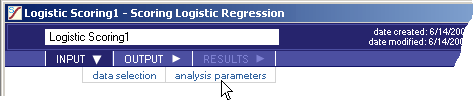# Logistic Scoring - INPUT - Analysis Parameters - Teradata Warehouse Miner

## Teradata Warehouse Miner User Guide - Volume 3Analytic Functions

Product
Release Number
5.4.5
Published
February 2018
Language
English (United States)
Last Update
2018-05-04
dita:mapPath
yuy1504291362546.ditamap
dita:ditavalPath
ft:empty
dita:id
B035-2302
Product Category
Software
1. On the Logistic Scoring dialog box, click INPUT.
2. Click analysis parameters.
Logistic Scoring > Input > Analysis Parameters3. On this screen, select:
• Scoring Method
• Score — Option to create a score table only.
• Evaluate — Option to perform model evaluation only.
• Evaluate and Score — Option to create a score table and perform model evaluation.
• Scoring Options
• Include Probability Score Column — Inclusion of a column in the score table that contains the probability between 0 and 1 that the value of the dependent variable is 1 is an optional parameter when scoring is selected. The default is to include a probability score column in the created score table. Either the probability score or the estimated value or both must be requested when scoring.
• Column Name — Column name containing the probability between 0 and 1 that the value of the dependent variable is 1.
• Include Estimate from Threshold Column — Inclusion of a column in the score table that contains the estimated value of the dependent variable is an option when scoring is selected. The default is to include an estimated value column in the created score table. Either the probability score or the estimated value or both must be requested when scoring.
• Column Name — Column name containing the estimated value of the dependent variable.
• Threshold Default — The threshold value is a value between 0 and 1 that determines which probabilities result in an estimated value of 0 or 1. For example, with a threshold value of 0.3, probabilities of 0.3 or greater yield an estimated value of 1, while probabilities less than 0.3 yield an estimated value of 0. The threshold option is valid only if the Include Estimate option has been requested and scoring is selected. If the Include Estimate option is requested but the threshold value is not specified, a default threshold value of 0.5 is used.
• Evaluation Options
• Prediction Success Table — Creates a prediction success table using sums of probabilities rather than estimates based on a threshold value. The default value is to include the Prediction Success Table. (This only applies if evaluation is requested).
• Multi-Threshold Success Table — This table provides values similar to those in the prediction success table, but based on a range of threshold values, thus allowing the user to compare success scenarios using different threshold values. The default value is to include the multi-threshold success table. (This only applies if evaluation is requested).
• Threshold Begin
• Threshold End
• Threshold Increment — Specifies the threshold values to be used in the multi-threshold success table. If the computed probability is greater than or equal to a threshold value, that observation is assigned a 1 rather than a 0. Default values are 0, 1 and .05, respectively.
• Cumulative Lift Table — Produce a cumulative lift table for deciles based on probability values. The default value is to include the cumulative lift table. (This only applies if evaluation is requested).